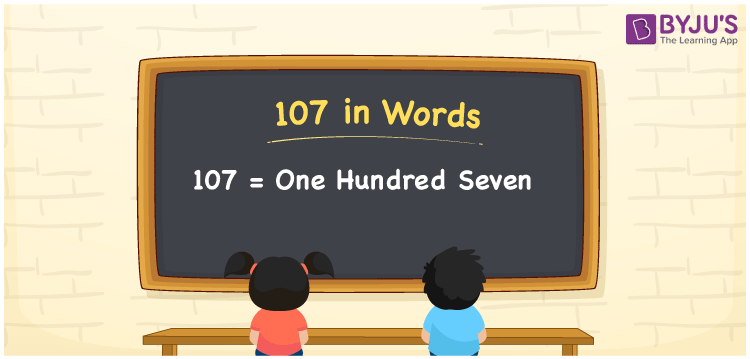# 107 in Words

107 in words is One hundred seven. In Mathematics, the number 107 is considered to be a cardinal number. For example, the cost of 1 kg apple is Rs. 107. In this article, let us have a look at the procedure of writing the number 107 in words using the place value system in detail.

 107 in Words: One Hundred Seven. One Hundred Seven in Numerical Form: 107.

## 107 in English Words## How to Write 107 in Words?

The number 107 is a three-digit number and its place values are provided below:

 Hundreds Tens Ones 1 0 7

The expanded form of 107 is as follows:

=1 × Hundred + 0 × Ten + 7 × One

=1 × 100 + 0 × 10 + 7 × 1

= 100 + 7

= 107

= One hundred seven

Hence, 107 in words is one hundred seven.

The number 107 appears after 106 but before 108.

107 in words – One hundred seven

Is 107 an odd number? – Yes

Is 107 an even number? – No

Is 107 a perfect square number? – No

Is 107 a perfect cube number? – No

Is 107 a prime number? – Yes

Is 107 a composite number? – No

## Frequently Asked Questions on 107 in Words

Q1

### Write 107 in words.

107 in words is one hundred seven.

Q2

### Simplify 100 + 7, and express it in words.

Simplifying 100 + 7, we get 107. Hence, 107 in words is one hundred seven.

Q3

### Is 107 a prime number?

Yes, 107 is a prime number.